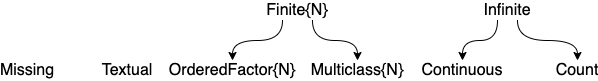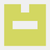## Julia Community 🟣# Julia Boards the Titanic- A brief introduction to the MLJ.jl package

This is a gentle introduction to Julia's machine learning toolbox MLJ focused on users new to Julia. In it we train a decision tree to predict whether a new passenger would survive a hypothetical replay of the Titanic disaster. The blog is loosely based on these notebooks.

Prerequisites: No prior experience with Julia, but you should know how to open a Julia REPL session in some terminal or console. A nodding acquaintance with supervised machine learning would be helpful.

Experienced data scientists may want to check out the more advanced tutorial, MLJ for Data Scientists in Two Hours.

## Decision Trees

Generally, decision trees are not the best performing machine learning models. However, they are extremely fast to train, easy to interpret, and have flexible data requirements. They are also the building blocks of more advanced models, such as random forests and gradient boosted trees, one of the most successful and widely applied class of machine learning models today. All these models are available in the MLJ toolbox and are trained in the same way as the decision tree.

Here's a diagram representing what a decision tree, trained on the Titanic dataset, might look like:For example, in this model, a male over the age of 9.5 is predicted to die, having a survival probability of 0.17.

## Package installation

We start by creating a new Julia package environment called `titanic`, for tracking versions of the packages we will need. Do this by typing these commands at the `julia>` prompt, with carriage returns added at the end of each line:

``````using Pkg
Pkg.activate("titanic", shared=true)
``````

To add the packages we need to your environment, enter the `]` character at the `julia>` prompt, to change it to `(titanic) pkg>`. Then enter:

``````add MLJ DataFrames BetaML
``````

It may take a few minutes for these packages to be installed and "precompiled".

Tip. Next time you want to use exactly the same combination of packages in a new Julia session, you can skip the `add` command and instead just enter the two lines above them.

When the `(titanic) pkg>` prompt returns, enter `status` to see the package versions that were installed. Here's what each package does:

• MLJ (machine learning toolbox): provides a common interface for interacting with models provided by different packages, and for automating common model-generic tasks, such as hyperparameter optimization demonstrated at the end of this blog.

• DataFrames: Allows you to manipulate tabular data that fits into memory. Tip. Checkout these cheatsheets.

• BetaML: Provides the core decision algorithm we will be building for Titanic prediction.

For now, return to the `julia>` prompt by pressing the "delete" or "backspace" key.

## Establishing correct data representation

``````using MLJ
import DataFrames as DF
``````

After entering the first line above we are ready to use any function in MLJ's documentation as it appears there. After the second, we can use functions from DataFrames, but must qualify the function names with a prefix `DF.`, as we'll see later.

In MLJ, and some other statistics packages, a "scientific type" or scitype indicates how MLJ will interpret data (as opposed to how it is represented on your machine). For example, while we have

``````julia> typeof(1)
Int64

julia> typeof(true)
Bool
``````

we have

``````julia> scitype(1)
Count
``````

but also

``````julia> scitype(true)
Count
``````

Tip. To learn more about a Julia command, use the `?` character. For example, try typing `?scitype` at the `julia>` prompt.

In MLJ, model data requirements are articulated using scitypes, which allows you to focus on what your data represents in the real world, instead of how it is stored on your computer.

Here are the most common "scalar" scitypes:We'll grab our Titanic data set from OpenML, a platform for sharing machine learning datasets and workflows. The second line below converts the downloaded data into a dataframe.

``````table = OpenML.load(42638)
df = DF.DataFrame(table)
``````

We can use DataFrames to get summary statistics for the features in our dataset:

``````DF.describe(df)
``````
Row variable mean min median max nmissing eltype
1 pclass 1 3 0 CategoricalValue{String, UInt32}
2 sex female male 0 CategoricalValue{String, UInt32}
3 age 29.7589 0.42 30.0 80.0 0 Float64
4 sibsp 0.523008 0.0 0.0 8.0 0 Float64
5 fare 32.2042 0.0 14.4542 512.329 0 Float64
6 cabin E31 C148 687 Union{Missing, CategoricalValue{…
7 embarked C S 2 Union{Missing, CategoricalValue{…
8 survived 0 1 0 CategoricalValue{String, UInt32}

In particular, we see that `cabin` has a lot of missing values, and we'll shortly drop it for simplicity.

To get a summary of feature scitypes, we use `schema`:

``````schema(df)
``````
Row names scitypes types
1 pclass Multiclass{3} CategoricalValue{String, UInt32}
2 sex Multiclass{2} CategoricalValue{String, UInt32}
3 age Continuous Float64
4 sibsp Continuous Float64
5 fare Continuous Float64
6 cabin Union{Missing, Multiclass{186}} Union{Missing, CategoricalValue{…
7 embarked Union{Missing, Multiclass{3}} Union{Missing, CategoricalValue{…
8 survived Multiclass{2} CategoricalValue{String, UInt32}

Now `sibsp` represents the number of siblings/spouses, which is not a continuous variable. So we fix that like this:

``````coerce!(df, :sibsp => Count)
``````

Call `schema(df)` again, to check a successful change.

## Splitting into train and test sets

To objectively evaluate the performance of our final model, we split off 30% of our data into a holdout set, called `df_test`, which will not used for training:

``````df, df_test = partition(df, 0.7, rng=123)
``````

You can check the number of observations in each set with `DF.nrow(df)` and `DF.nrow(df_test)`.

## Splitting data into input features and target

In supervised learning, the target is the variable we want to predict, in this case `survived`. The other features will be inputs to our predictor. The following code puts the `df` column with name `survived` into the vector `y` (the target) and everything else, except `cabin`, which we're dropping, into a new dataframe called `X` (the input features).

``````y, X = unpack(df, ==(:survived), !=(:cabin));
``````

You can check `X` and `y` have the expected form by doing `schema(X)` and `scitype(y)`.

We'll want to do the same for the holdout test set:

``````y_test, X_test = unpack(df_test, ==(:survived), !=(:cabin));
``````

## Choosing a supervised model:

There are not many models that can directly handle missing values and a mixture of scitypes, as we have here. Here's how to list the ones that do:

``````julia> models(matching(X, y))
(name = ConstantClassifier, package_name = MLJModels, ... )
(name = DecisionTreeClassifier, package_name = BetaML, ... )
(name = DeterministicConstantClassifier, package_name = MLJModels, ... )
(name = RandomForestClassifier, package_name = BetaML, ... )
``````

This shortcoming can be addressed with data preprocessing provided by MLJ but not covered here, such as one-hot encoding and missing value imputation. We'll settle for the indicated decision tree.

The code for the decision tree model is not available until we explicitly load it, but we can already inspect it's documentation. Do this by entering `doc("DecisionTreeClassifier", pkg="BetaML")`. (To browse all MLJ model documentation use the Model Browser.)

An MLJ-specific method for loading the model code (and necessary packages) is shown below:

``````Tree = @load DecisionTreeClassifier pkg=BetaML
tree = Tree()
``````

The first line loads the model type, which we've called `Tree`; the second creates an object storing default hyperparameters for a `Tree` model. This `tree` will be displayed thus:

``````DecisionTreeClassifier(
max_depth = 0,
min_gain = 0.0,
min_records = 2,
max_features = 0,
splitting_criterion = BetaML.Utils.gini,
rng = Random._GLOBAL_RNG())
``````

We can specify different hyperparameters like this:

``````tree = Tree(max_depth=10)
``````

## Training the model

We now bind the data to be used for training and the hyperparameter object `tree` we just created in a new object called a machine:

``````mach = machine(tree, X, y)
``````

We train the model on all bound data by calling `fit!` on the machine. The exclamation mark `!` in `fit!` tells us that `fit!` mutates (changes) its argument. In this case the model's learned parameters (the actual decision tree) is stored in the `mach` object:

``````fit!(mach)
``````

Before getting predictions for new inputs, let's start by looking at predictions for the inputs we trained on:

``````p = predict(mach, X)
``````

Notice that these are probabilistic predictions. For example, we have

``````julia> p
UnivariateFinite{Multiclass{2}}
┌                                        ┐
0 ┤■■■■■■■■■■■■■■■■■■■■■■■■■■■■■■ 0.914894
1 ┤■■■ 0.0851064
└                                        ┘
``````

Extracting a raw probability requires an extra step. For example, to get the survival probability (`1` corresponding to survival and `0` to death), we do this:

``````julia> pdf(p, "1")
0.0851063829787234
``````

We can also get "point" predictions using the `mode` function and Julia's broadcasting syntax:

``````julia> yhat = mode.(p)
julia> yhat[3:5]
3-element CategoricalArrays.CategoricalArray{String,1,UInt32}:
"0"
"0"
"1"
``````

## Evaluating model performance

Let's see how accurate our model is at predicting on the data it trained on:

``````julia> accuracy(yhat, y)
0.921474358974359
``````

Over 90% accuracy! Better check the accuracy on the test data that the model hasn't seen:

``````julia> yhat = mode.(predict(mach, X_test));
julia> accuracy(yhat, y_test)
0.7790262172284644
``````

Oh dear. We are most likely overfitting the model. Still, not a bad first step.

The evaluation we have just performed is known as holdout evaluation. MLJ provides tools for automating such evaluations, as well as more sophisticated ones, such as cross-validation. See this simple example and the detailed documentation for more information.

## Tuning the model

Changing any hyperparameter of our model will alter it's performance. In particular, changing certain parameters may mitigate overfitting.

In MLJ we can "wrap" the model to make it automatically optimize a given hyperparameter, which it does by internally creating its own holdout set for evaluation (or using some other resampling scheme, such as cross-validation) and systematically searching over a specified range of one or more hyperparameters. Let's do that now for our decision tree.

First, we define a hyperparameter range over which to search:

``````r = range(tree, :max_depth, lower=0, upper=8)
``````

Note that, according to the document string for the decision tree (which we can retrieve now with `?Tree`) we see that `0` here means "no limit on `max_depth`".

Next, we apply MLJ's `TunedModel` wrapper to our tree, specifying the range and performance measure to use as a basis for optimization, as well as the resampling strategy we want to use, and the search method (grid in this case).

``````tuned_tree = TunedModel(
tree,
tuning = Grid(),
range=r,
measure = accuracy,
resampling=Holdout(fraction_train=0.7),
)
``````

The new model `tuned_tree` behaves like the old, except that the `max_depth` hyperparameter effectively becomes a learned parameter instead.

Training this `tuned_tree` actually performs two operations, under the hood:

• Search for the best model using an internally constructed holdout set

• Retrain the "best" model on all available data

``````mach2 = machine(tuned_tree, X, y)
fit!(mach2)
``````

Here's how we can see what the optimal model actually is:

``````julia> fitted_params(mach2).best_model
DecisionTreeClassifier(
max_depth = 6,
min_gain = 0.0,
min_records = 2,
max_features = 0,
splitting_criterion = BetaML.Utils.gini,
rng = Random._GLOBAL_RNG())
``````

Finally, let's test the self-tuning model on our existing holdout set:

``````yhat2 = mode.(predict(mach2, X_test))
accuracy(yhat2, y_test)
0.8164794007490637
``````

Although we cannot assign this outcome statistical signicance, without a more detailed analysis, this appears to be an improvement on our original `depth=10` model.

## Learning more

Suggestions for learning more about Julia and MLJ are here.